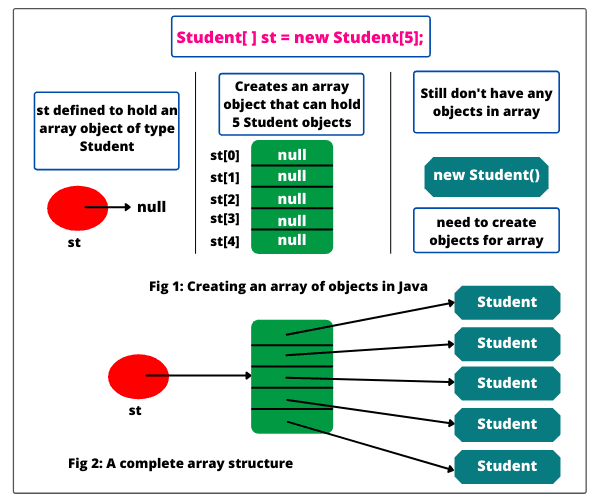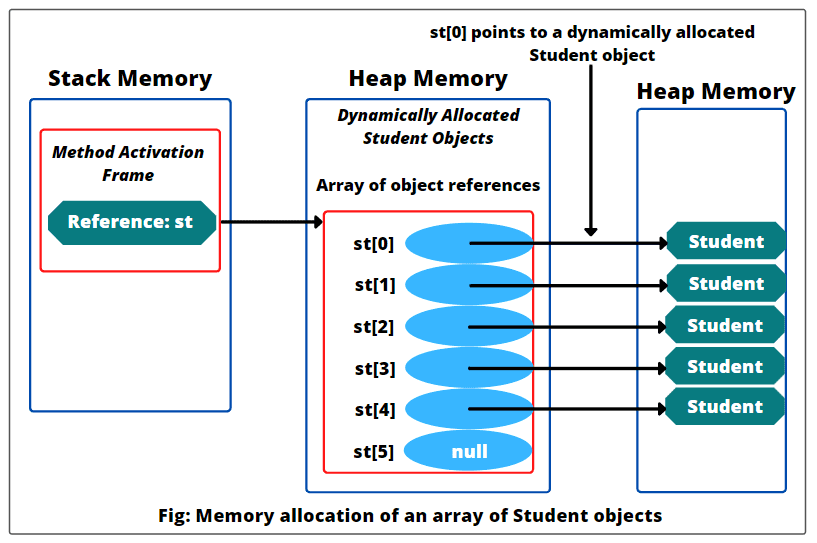# Arrays of Objects in Java | Example Program

Arrays of Objects in Java | So far, we have studied in the previous tutorial that an array in java is an ordered, sequential group of elements. These elements can be either primitive types or reference types.

The main difference between arrays of primitive types and arrays of reference types is that primitive type values can be directly assigned to elements of primitive type arrays.

But, it does not happen in reference-type elements. In the case of an array of reference types, each element contains a reference (normally address ) to an object in the memory.

When we create an array of references in memory, actually, we are not automatically creating an object of each element.

Each reference element is automatically initialized to null and the object that we want it to point to must be explicitly built. (Or the object must already exist somewhere in the memory and be accessible).

To demonstrate these concepts we will understand arrays of objects in java in detail.

## Arrays of Objects in Java

An array of objects in Java is just a list of reference variables that contain normally addresses to the individual objects.

When arrays of objects are allocated in the heap memory, only the references for objects are created, no objects are actually created. The actual objects to be stored must be allocated individually at runtime.

In other simple words, for arrays of objects, an object must be created separately for each element in the array.

Let’s understand the concepts of an array of objects in java with help of examples more clearly.

Suppose we have a class Student and we have created an array of students.

```Student[ ] st = new Student; // An array of objects of length 5 is declared and created.
```

Let’s understand this statement in three steps with a diagram.

Step 1: Declare a Student array variable.

```Student[ ] st;
```

Here, we have declared Student array variable, that can hold a reference to an array object of Students. At present, this array is empty does not hold references to any instances of Student.

Step 2: After declaring Student array variable, the next step is to create Student array object with a length of 5 by using the new operator, and assign it to that Student variable st.

```st = new Student;
```

It creates an array capable of holding 5 Student objects. When we create an array of objects using new operator in java, all its slots automatically are initialized with null.

Note: Java keyword null refers to null object and can be used for any object reference.

What’s missing?

Student! We have an array of Student references, but no actual Student objects.

Step 3: Now, create new Student objects, and assign them to the array elements like this:

```st = new Student();
st = new Student();
st = new Student();
st = new Student();
st = new Student();
```

Here, five new Student objects are created and their memory location is assigned to the Student reference located in st, st, st, st, and st, respectively. Look at the below figure to understand better.The above figure shows that the creation of an array of objects in java consists of three-stage processes that are as follows:

1. Create an array variable that can reference an array of appropriate types of objects.

2. Create an array of objects.

3. Fill the array of objects with instances of the appropriate type.

## Example Program based on Array of Objects

1. Let’s take a very simple example program where we will create an array of Student objects of length 5 and assign new Student objects to the array elements. Look at the source code below to understand more clearly.

Program code:

```package arraysProgram;
public class Student
{
String name;
int rollNo;
Student(String name, int rollNo)
{
this.name = name;
this.rollNo = rollNo;
}
}
package arraysProgram;
public class ArraysOfObjects {
public static void main(String[] args)
{
// Creating an array of Student objects of length 5.
Student[ ] st = new Student;
// Assigning new Student objects to the array elements.
st = new Student("John", 1);
st = new Student("Ivaan", 2);
st = new Student("Deepak", 3);
st = new Student("Amit", 4);
st = new Student("Rashmi", 5);
st = new Student("Herry", 6);

// Accessing elements of the specified array of objects using array references.
for(int i = 0; i < st.length; i++)
{
System.out.println("Name: " +st[i].name+ ", "+"Roll no: " +st[i].rollNo);
}
}
}
```
```Output:
Name: John, Roll no: 1
Name: Ivaan, Roll no: 2
Name: Deepak, Roll no: 3
Name: Amit, Roll no: 4
Name: Rashmi, Roll no: 5
Name: Herry, Roll no: 6
```

Creating an array of Student objects and assigning new Student objects to the array elements can also be done like this:

```Student[ ] st = new Student[ ]
{
new Student("John", 1);
new Student("Ivaan", 2);
new Student("Deepak", 3);
new Student("Amit", 4);
new Student("Rashmi", 5);
new Student("Herry", 6);
};
```

Look at the below figure to understand the memory allocation of an array of Student objects.In this program, we have declared and created an array of Student objects of length 6. As shown in the above figure, object reference variable st contains an address that is stored in the stack memory.

This reference st will point to an array of Student object references in the heap memory that is initialized with null initially.

When we have created instances of Student object, its memory location is created in heap and is assigned to the array of object references stored in heap memory. For example, st now points to a dynamically allocated Student object.

2. Let’s create another program based on the arrays of objects in java. In this program, we will create an array of School objects and fill array objects with instances of School object.

After initialization, we will send different values to variables declared in School class by using array references for separate School objects and then display them on the console.

Program code:

```package arraysProgram;
public class School
{
String stName;
String scName;
int rollNo;
double per;
void display()
{
System.out.println(stName+ " " +scName+ " " +rollNo+ " " +per+ "%");
}
}
package arraysProgram;
public class SchoolTestDrive {
public static void main(String[ ] args)
{
// Create a School array.
School[ ] sc = new School;
// Put some School into it.
sc = new School();
sc = new School();
sc = new School();

// Accessing School using array references.
sc.stName = "Ivaan";
sc.stName = "Deepak";
sc.stName = "Herry";
sc.scName = "RSVM";
sc.scName = "DPS";
sc.scName = "DAV";
sc.rollNo = 2;
sc.rollNo = 5;
sc.rollNo = 8;
sc.per = 98.8;
sc.per = 99;
sc.per = 97.5;

int x = 0;
while(x < sc.length)
{
sc[x].display();
x = x + 1;
}
}
}
```
```Output:
Ivaan RSVM 2 98.8%
Deepak DPS 5 99.0%
Herry DAV 8 97.5%
```

3. Let’s create a program based on an array of objects in which we will store objects of three classes A, B, and C into it and access methods of classes A, B, and C using their array references.

Program code:

```public class A {
int x;
A(int x) {
this.x = x;
}
void m1() {
System.out.println("Value of x: " +x);
}
}
public class B {
int y;
B(int y) {
this.y = y;
}
void m2(){
System.out.println("Value of y: " +y);
}
}
public class C {
int z;
C(int z) {
this.z = z;
}
void m3(){
System.out.println("Value of z: "+z);
}
}
public class ArrayObjects {
public static void main(String[] args)
{
A[ ] a = new A;
B[ ] b = new B;
C[ ] c = new C;
a = new A(25);
b = new B(30);
c = new C(35);

a.m1();
b.m2();
c.m3();
}
}
```
```Output:
Value of x: 25
Value of y: 30
Value of z: 35
```

In the above program 3, we have declared and created arrays of objects of three different classes A, B, and C of the same length 1 and then assigned arrays of objects with instances of classes A, B, and C. We have easily accessed methods of three classes using array references.

Now, we will modify something in this program. Now, we will declare and create an array of Object objects of length 3 and assign array objects with instances of three classes A, B, and C.

But in this program, we need to do type casting to access methods of these classes. Look at the source code to understand better.

Program code:

```public class A {
public class ArrayObjects {
public static void main(String[] args)
{
// Creating an array of Objects of length 3.
Object[ ] obj = new Object;
// Putting three objects of different data types.
obj = new A(20);
obj = new B(40);
obj = new C(50);

((A) obj).m1(); // Type casting.
((B) obj).m2();
((C) obj).m3();
}
}
```
```Output:
Value of x: 20
Value of y: 40
Value of z: 50
```

Program code: For practice and enhance your knowledge.

```public class X {
String m1() {
System.out.println("m1-X");
return null;
}
}
public class Y {
Object m2(){
System.out.println("m2-Y");
return null;
}
}
public class ArrayObjects {
public static void main(String[] args)
{
// Creating an array of Objects of length 2.
Object[ ] obj = new Object;
obj = new X();
obj = new Y();
obj = new Object();

((X) obj).m1();
((Y) obj).m2();
System.out.println(obj);
}
}
```
```Output:
m1-X
m2-Y
[email protected]

```

Key points associated with arrays of Objects in Java:

1. Arrays are always objects in java whether we declare to hold primitives or object references.

2. A reference variable can be used to access a method or instance variable using dot operator (.).

3. An object reference variable has a value of null when it is not referencing any object.

4. An array of object references can take objects of any type.

5. Java compiler cannot determine which objects are stored in an array.

6. Several objects of different data types (such as an Object, Person, or Car) added in an array cane only be determined at runtime by JVM.

Hope that this tutorial has explained almost all the important points associated with arrays of objects in java with different example programs. I hope that you will have understood the basic concepts of java arrays of objects.

If you get anything incorrect in this tutorial, then please inform our team via email. Your email will be precious to us.